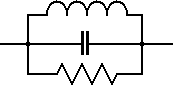# Capacitor Inductor and Resistor in Parallel Calculator

This calculator finds the complex impedance (real and imaginary imaginary values) of a capacitor, inductor and resistor in parallel.Frequency: MHz kHz Hz Capacitance: uF nF pF Inductance: mH uH nH Resistance: MΩ kΩ Ω Z =

The complex impedance (Z) (real and imaginary) of a capacitor, inductor and a resistor in parallel at a particular frequency can be calculated using the following equations.Where:

f is the Frequency in Hz

C is the Capacitance in Farads

L is the Inductance in Henries

R is the Resistance in Ohms

XC is the Capacitive Reactance in Ohms

XL is the Inductive Reactance in Ohms

XT is the Total Reactance in Ohms

This calculator uses JavaScript and will function in most modern browsers. For more information see About our calculators

This calculator is provided free by Chemandy Electronics in order to promote the FLEXI-BOX